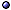Differential Equations

In General
* History: Originated with the invention of calculus (Newton and Leibniz, 1670–1680); They were first used to solve geometrical problems, then dynamical ones starting with Euler around 1730.
* Order: The highest derivative appearing in the equation.
* Degree: The highest power to which the highest derivative is raised, if the differential equation is polynomial.
* Varying the equation: "Close differential equations give close solutions", but this closeness is not uniform over t; The solutions almost never stay close, and the trajectories, as sets, may or may not be close; > s.a. chaos.
@ General references: Vrabie 16 [intro text]; Székelyhidi 16 [intro text].
@ Existence, uniqueness, regularity: in Gallavotti 83.
@ Symmetries: Krasil'shchik & Vinogradov 98 [and conservation laws]; Duarte & da Mota a1007 [semi-algorithmic method for finding Lie symmetries]; Peng a2009 [modified formal Lagrangian formulation for general differential equations].
@ And topology: Filippov 98.
@ Related topics: Gitman & Kupriyanov JPA(07)-a0710 [action principle]; > s.a. Variational Principles.

Types and Solution Methods > s.a. Boundary-Value Problems; Darboux Transformation; ordinary differential equations; partial differential equations.
* Numerical: A robust one is the Runge-Kutta method (errors can only grow at a polynomial rate).
@ General references: Titchmarsh 62 [eigenfunction expansion]; Ablinger et al a1601 [coupled systems]; Loustau 16 [numerical].
@ Integrable: Bracken a0903 [and theory of surfaces].
@ Stochastic: Burrage et al PRS(04) [numerical]; Williams PhyA(08) [stochastic Ansatz for solution]; > s.a. partial differential equations.
@ Discretization: Murata et al JPA(10) [systematic, for first-order equations]; Bihlo JPA(13)-a1210 [invariant meshless discretization schemes]; Campoamor-Stursberg et al JPA(16)-a1507 [for ODEs, symmetry preserving]; > s.a. Difference Equations.
@ Runge-Kutta method: Kalogiratou et al PRP(14); O'Sullivan JPCS(17)-a1702 [Factorized Runge-Kutta-Chebyshev methods]; Tapley a2105 [preservation of second integrals]; > s.a. computational physics.
@ Other types: de Gosson et al mp/00-proc, Kedlaya 10 [p-adic]; Maj mp/04-proc [pseudo-differential wave equations]; Carlsson et al a1403 [linear differential equations with infinitely many derivatives]; Grabowski & de Lucas a1810 [superdifferential equations]; > functional analysis; p-Adic Numbers; quaternions.

Integro-Differential Equations
* Idea: Typical examples include time-dependent diffusion equations containing a parameter (e.g., the temperature) that depends on integrals of the unknown distribution function; The standard approach to solving the resulting non-linear pde involves the use of iterative predictor-corrector algorithms.
@ General references: Becker JMP(99) [perturbative approach]; Dattoli et al NCB(04) [operator method].
@ Applications: Tarasov TMP(09)-a1107 [fractional, electromagnetic waves in a dielectric].

Fractional Order Differential Equations > s.a. diffusion; fractional calculus; fractals.
@ Books: Kilbas et al 06; Guo et al 15 [and numerical solutions]; Zhou et al 16 [basic theory].
@ General references: Gorenflo & Mainardi in(97)-a0805; Ciesielski & Leszczynski mp/03-conf [and anomalous diffusion]; Duan JMP(05) [time and space]; Kochubei FCAA-a0806 [solutions and singularities]; Podlubny et al JCP(09) [matrix approach]; Wu a1006 [variational approach]; Saxena et al a1109 [computable solutions]; Leo et al CRM(14)-a1402, a1405 [symmetries].
@ Specific types of equations: Saxena et al EJPAM-a1406 [analytical solutions of fractional order Laplace, Poisson and Helmholtz equations in two variables].
@ And brownian motion: Mainardi & Pironi EM(96)-a0806; Lim & Muniandy PLA(00); Hochberg & Pérez-Mercader PLA(02) [and renormalization]; McCauley et al PhyA(07) [vs Gaussian Markov processes, and Hurst exponents]; Duarte & Guimarães PLA(08) [and fractional derivatives]; > s.a. brownian motion.
@ Fractional quantum mechanics / Schrödinger equation: Laskin PRE(02)qp; Naber JMP(04)mp [time-fractional]; Herrmann mp/05; Guo & Xu JMP(06) [examples]; Iomin PRE(09)-a0909; Jeng et al JMP(10) [non-locality]; Laskin a1009 [rev].
@ Other applications: Hilfer ed-00 [in physics]; Klafter et al ed-11; Tarasov TMP(09)-a1107 [electromagnetic fields in dielectric media].Specific types of equations: see fokker-planck equation; poisson equation.